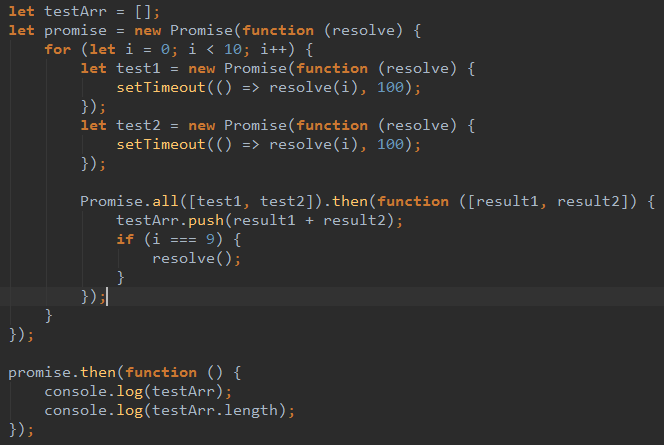js在for循环中执行异步操作，把每次异步的结果保存在数组中，如何在循环外面使用数组？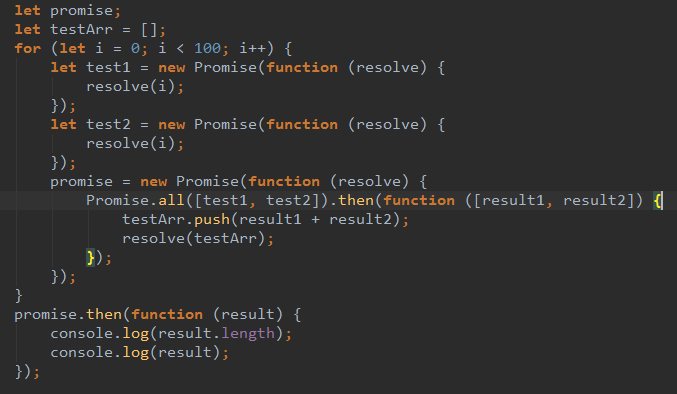0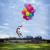1、Promise.All

``````
function asyncRandom() {
return new Promise(function(resolve, reject) {
setTimeout(resolve, 100, Math.floor(Math.random() * 100) + 1);
});
}

let numbers = [];
function genNumber(count = 100) {
const promises = [];
const handleNumber = n => numbers.push(n);
for (let i = 0; i < count; i++) {
promises.push(asyncRandom().then(handleNumber));
}
return promises;
}

Promise.all(genNumber(20)).then(() => {
console.log(numbers);
});``````

2、async function（这种是一个一个处理的，每个promise处理完再处理下一个，相当于同步的方案，执行效率低）

``````function asyncRandom() {
return new Promise(function(resolve, reject) {
setTimeout(resolve, 100, Math.floor(Math.random() * 100) + 1);
});
}

async function getManyNumbers(count=100) {
let result = [];//也可以定义在外部

for (let i = 0; i < count; i++) {
const ret = await asyncRandom();
result.push(ret);
}
return result;
}

getManyNumbers().then(numbers => console.log(numbers));

// getManyNumbers().then(() => console.log(numbers)); // 定义在外部时``````

3、es7 generator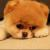0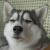0